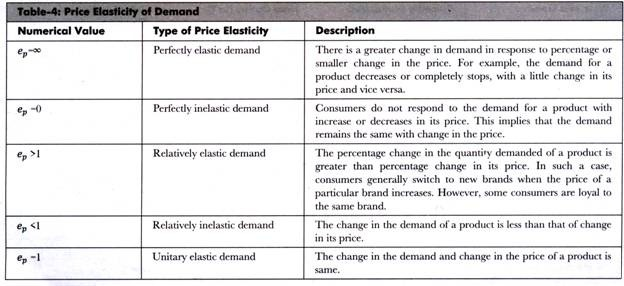# 5 Types of Price Elasticity of Demand – Explained!

The extent of responsiveness of demand with change in the price is not always the same.

The demand for a product can be elastic or inelastic, depending on the rate of change in the demand with respect to change in price of a product.

Elastic demand is the one when the response of demand is greater with a small proportionate change in the price. On the other hand, inelastic demand is the one when there is relatively a less change in the demand with a greater change in the price.

For better understanding the concepts of elastic and inelastic demand, the price elasticity of demand has been divided into five types, which are shown in Figure-1: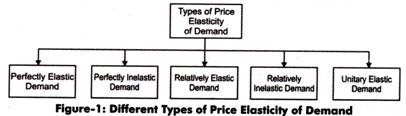Let us discuss the different types of price elasticity of demand (as shown in Figure-1).

### 1. Perfectly Elastic Demand:

When a small change in price of a product causes a major change in its demand, it is said to be perfectly elastic demand. In perfectly elastic demand, a small rise in price results in fall in demand to zero, while a small fall in price causes increase in demand to infinity. In such a case, the demand is perfectly elastic or ep = 00.

The degree of elasticity of demand helps in defining the shape and slope of a demand curve. Therefore, the elasticity of demand can be determined by the slope of the demand curve. Flatter the slope of the demand curve, higher the elasticity of demand.

In perfectly elastic demand, the demand curve is represented as a horizontal straight line, which is shown in Figure-2: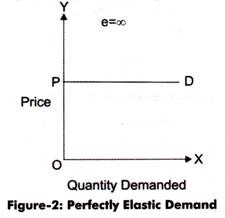From Figure-2 it can be interpreted that at price OP, demand is infinite; however, a slight rise in price would result in fall in demand to zero. It can also be interpreted from Figure-2 that at price P consumers are ready to buy as much quantity of the product as they want. However, a small rise in price would resist consumers to buy the product.

Though, perfectly elastic demand is a theoretical concept and cannot be applied in the real situation. However, it can be applied in cases, such as perfectly competitive market and homogeneity products. In such cases, the demand for a product of an organization is assumed to be perfectly elastic.

From an organization’s point of view, in a perfectly elastic demand situation, the organization can sell as much as much as it wants as consumers are ready to purchase a large quantity of product. However, a slight increase in price would stop the demand.

### 2. Perfectly Inelastic Demand:

A perfectly inelastic demand is one when there is no change produced in the demand of a product with change in its price. The numerical value for perfectly inelastic demand is zero (ep=0).

In case of perfectly inelastic demand, demand curve is represented as a straight vertical line, which is shown in Figure-3: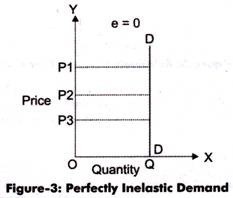It can be interpreted from Figure-3 that the movement in price from OP1 to OP2 and OP2 to OP3 does not show any change in the demand of a product (OQ). The demand remains constant for any value of price. Perfectly inelastic demand is a theoretical concept and cannot be applied in a practical situation. However, in case of essential goods, such as salt, the demand does not change with change in price. Therefore, the demand for essential goods is perfectly inelastic.

### 3. Relatively Elastic Demand:

Relatively elastic demand refers to the demand when the proportionate change produced in demand is greater than the proportionate change in price of a product. The numerical value of relatively elastic demand ranges between one to infinity.

Mathematically, relatively elastic demand is known as more than unit elastic demand (ep>1). For example, if the price of a product increases by 20% and the demand of the product decreases by 25%, then the demand would be relatively elastic.

The demand curve of relatively elastic demand is gradually sloping, as shown in Figure-4:It can be interpreted from Figure-4 that the proportionate change in demand from OQ1 to OQ2 is relatively larger than the proportionate change in price from OP1 to OP2. Relatively elastic demand has a practical application as demand for many of products respond in the same manner with respect to change in their prices.

For example, the price of a particular brand of cold drink increases from Rs. 15 to Rs. 20. In such a case, consumers may switch to another brand of cold drink. However, some of the consumers still consume the same brand. Therefore, a small change in price produces a larger change in demand of the product.

### 4. Relatively Inelastic Demand:

Relatively inelastic demand is one when the percentage change produced in demand is less than the percentage change in the price of a product. For example, if the price of a product increases by 30% and the demand for the product decreases only by 10%, then the demand would be called relatively inelastic. The numerical value of relatively elastic demand ranges between zero to one (ep<1). Marshall has termed relatively inelastic demand as elasticity being less than unity.

The demand curve of relatively inelastic demand is rapidly sloping, as shown in Figure-5: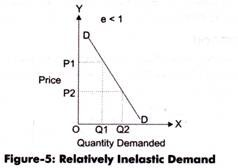It can be interpreted from Figure-5 that the proportionate change in demand from OQ1 to OQ2 is relatively smaller than the proportionate change in price from OP1 to OP2. Relatively inelastic demand has a practical application as demand for many of products respond in the same manner with respect to change in their prices. Let us understand the implication of relatively inelastic demand with the help of an example.

Example-3:

The demand schedule for milk is given in Table-3:

Calculate the price elasticity of demand and determine the type of price elasticity.

Solution:

P= 15

Q = 100

P1 = 20

Q1 = 90

Therefore, change in the price of milk is:

∆P = P1 – P

∆P = 20 – 15

∆P = 5

Similarly, change in quantity demanded of milk is:

∆Q = Q1 – Q

∆Q = 90 – 100

∆Q = -10

The change in demand shows a negative sign, which can be ignored. This is because of the reason that the relationship between price and demand is inverse that can yield a negative value of price or demand.

Price elasticity of demand for milk is:

ep = ∆Q/∆P * P/Q

ep = 10/5 * 15/100

ep = 0.3

The price elasticity of demand for milk is 0.3, which is less than one. Therefore, in such a case, the demand for milk is relatively inelastic.

### 5. Unitary Elastic Demand:

When the proportionate change in demand produces the same change in the price of the product, the demand is referred as unitary elastic demand. The numerical value for unitary elastic demand is equal to one (ep=1).

The demand curve for unitary elastic demand is represented as a rectangular hyperbola, as shown in Figure-6:

From Figure-6, it can be interpreted that change in price OP1 to OP2 produces the same change in demand from OQ1 to OQ2. Therefore, the demand is unitary elastic.

The different types of price elasticity of demand are summarized in Table-4: